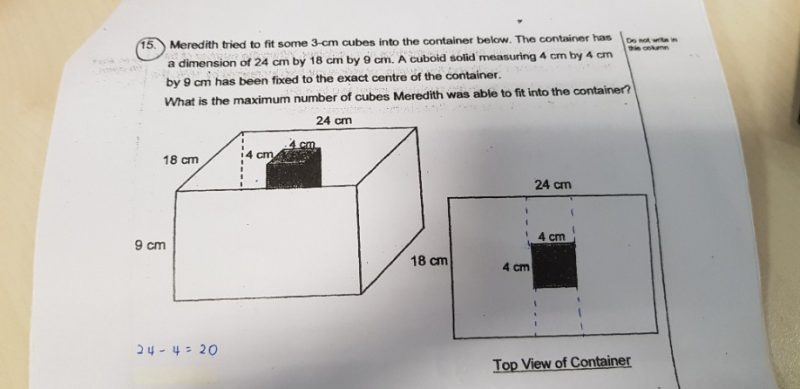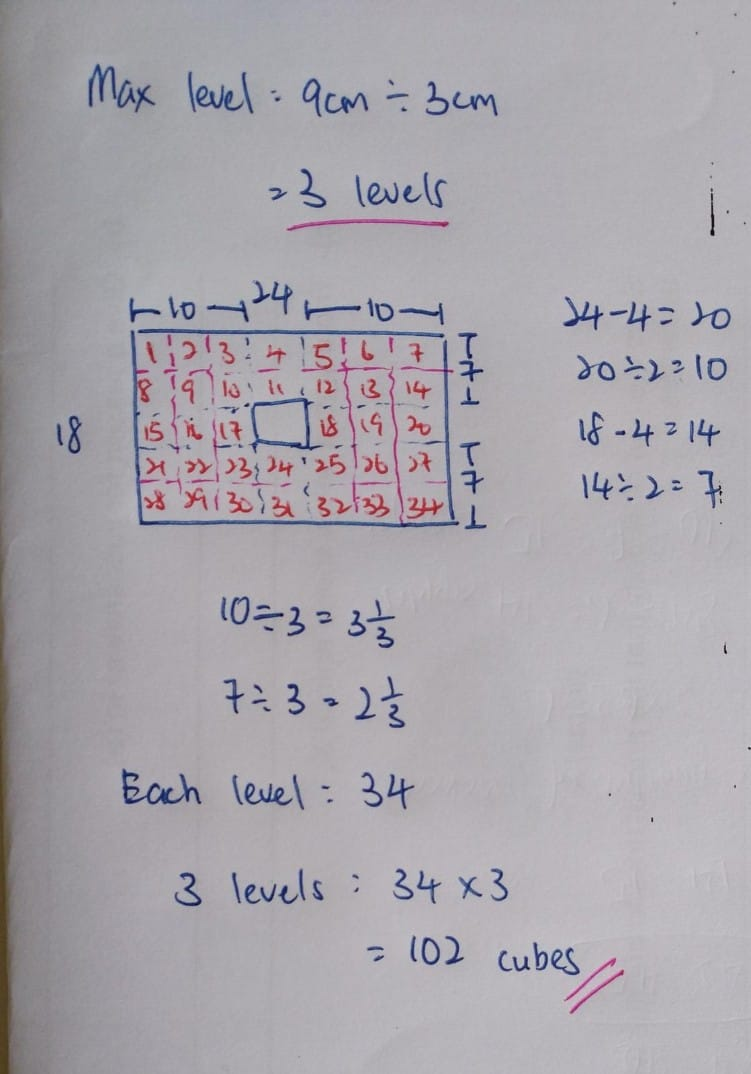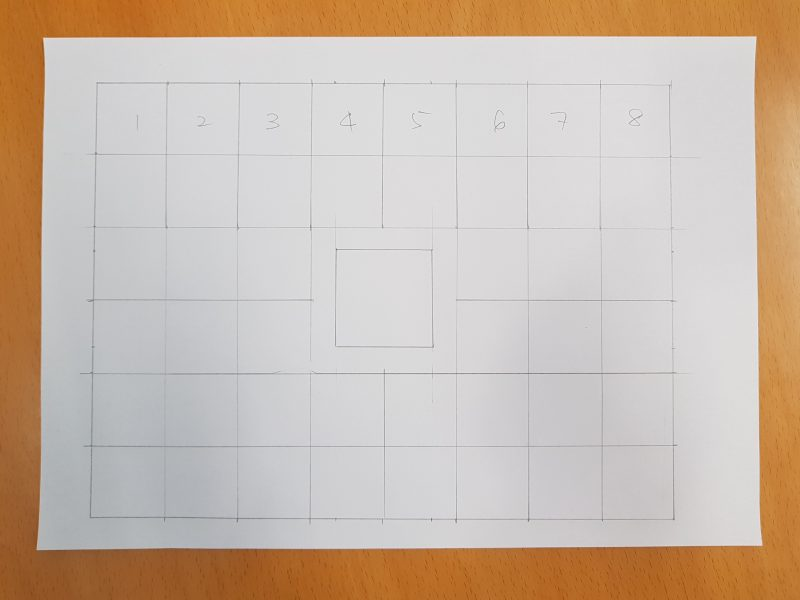# QuestionHello, can i get help for this question pls. ThanksThe answer key is 138. I’m not sure how they got 138 either.

2 Replies 0 Likes

24 x 18 x 9 = 3888

4 x 4 x 9 = 144

3888 – 144 = 3744

3 x 3 x 3 = 27

3744/27 = 138 (rounded down to the nearest whole number)

Please note that the above solution is, however, incorrect as there are spaces that 6 (3 cm by 3 cm by 3 cm) cubes could not fit in.

0 Replies 0 Likes

I got 114.

I think the answer is 120.

Because I made a mistake at the side. If you notice the top view is 18 cm, it could fit in 6 cubes.
So, on each side of the 10 cm, can fit in one more row, making it 18 cubes on each side. Plus, the 4 cubes in the middle column, each level can fit in 18 + 18 + 4 = 40 cubes.

Therefore, there are 3 level (as the height is 9 cm),
it will be
40 cubes x 3 levels = 120 cubes.

0 Replies 0 Likes

Method 1 :
24/3 = 8
18 – 4 = 14
14/2 = 7
7/3 = 2R1
9/3 = 3
8 x 2 x 3 x 2 = 96
24 – 4 = 20
20/2 = 10
10/3 = 3R1
18 – (3 x 2 x 2) = 6
6/3 = 2
9/3 = 3
3 x 2 x 3 x 2 = 36
96 + 36 = 132

Method 2 :
18/3 = 6
24 – 4 = 20
20/2 = 10
10/3 = 3
9/3 = 3
6 x 3 x 3 x 2 = 108
18 – 4 = 14
14/2 = 7
7/3 = 2R1
24 – (3 x 3 x 2) = 6
6/3 = 2
9/3 = 3
2 x 2 x 3 x 2 = 24
108 + 24 = 132

Ans : 132 cubes.

0 Replies 0 LikesTop portion of the cuboid

No of squares = 24×6/9 = 16

Lower portion of the cuboid

No of squares = 24×6/9 = 16

Left side of the cuboid

Length = (24-4)/2 = 10

Breath = 18-6-6 = 6

Hence, no of squares = 9×6/9 = 6

This is the same for right side of the cuboid, which is also 6 squares.

Height of this container = 9cm

Cube’s height is 3cm, we can have three layers.

one layer is 16+16+6+6 = 44 (cubes)

three layers = 132 (cubes)

0 Replies 0 Likes